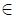Competitions

# ABCDEF

Time limit 1 second
Memory limit 128 MiB

You are given a set S of integers between -30000 and 30000 (inclusive).Find the total number of sixtuples (a, b, c, d, e, f) : a, b, c, d, e, f S, d0 that satisfy:

(a * b + c) / d - e = f

## Input data

The first line contains integer n (1n100), the size of a set S. Elements of S are given in the second line. Given numbers will be distinct.

## Output data

Print the total number of plausible sixtuples.

## Examples

Input example #1
3
5 7 10

Output example #1
10